# Fundamentals of image convolutions

Updated: Dec 23, 2019

### Fundamentals of image convolutions

Image convolution is powerful technique of modifying image by convolving a small 3x5, 5x5 matrix called kernel with image to product effects like emboss, outline, blur, sharpen. Convolving involves sliding a kernel over original image from left to right and top to bottom one row and column at a time, doing element wise multiplication and then summing the result. Depending on the values uses in the kernel, the outcome varies.

While human enjoys aesthetics of Pittsburg skyline on the left below, a machine sees black & white image just as array of pixel intensities on the right.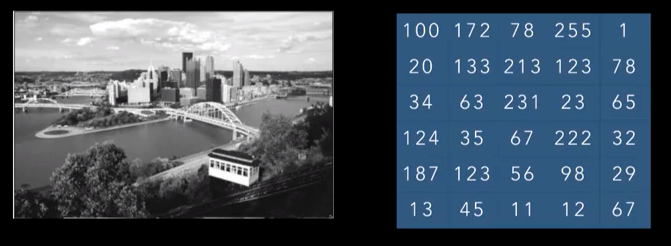A convolution operation involves taking a small matrix like 3x3 shown below on the right, and slide it on original image matrix from left to right and top to beootom and at each iteration, perform element wise multiplication and then take a sum.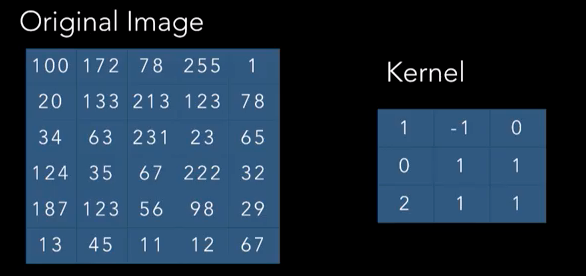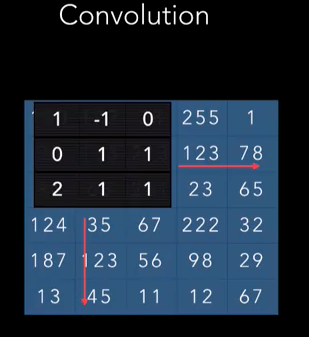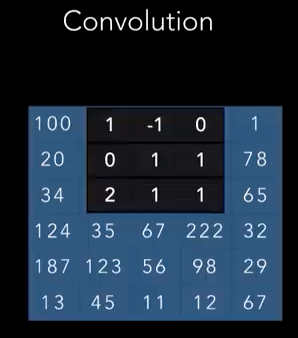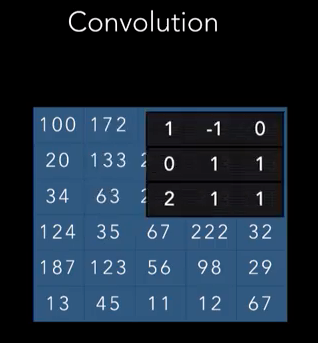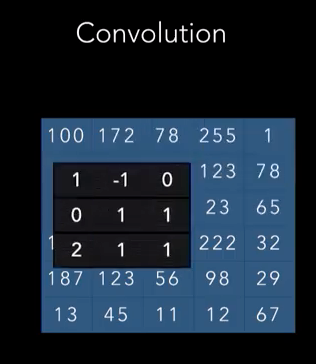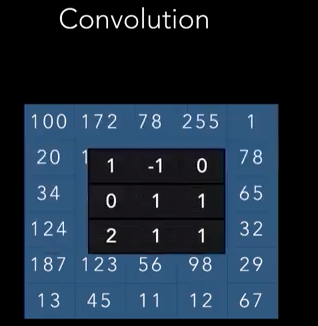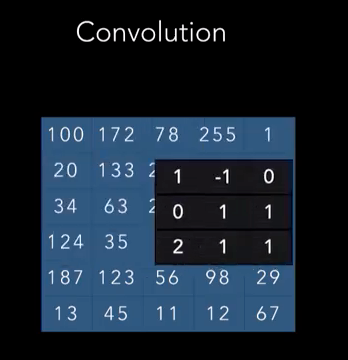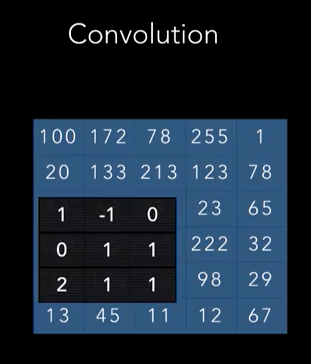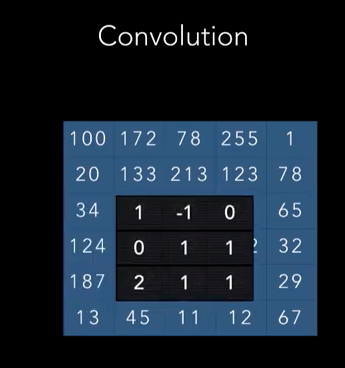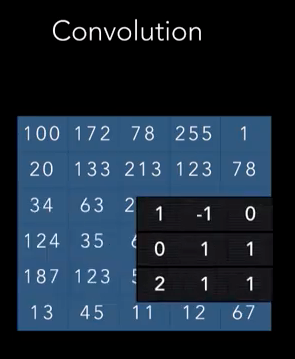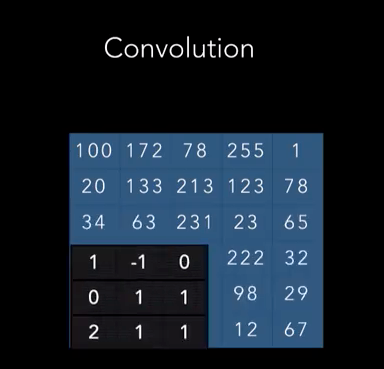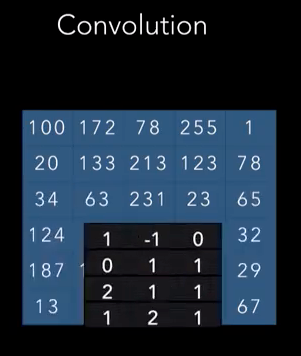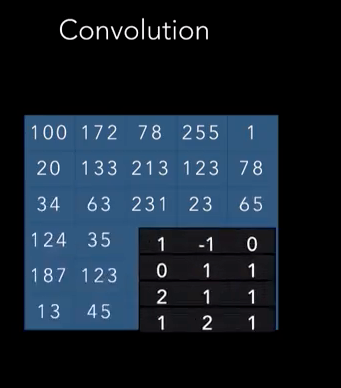One convolution Operation: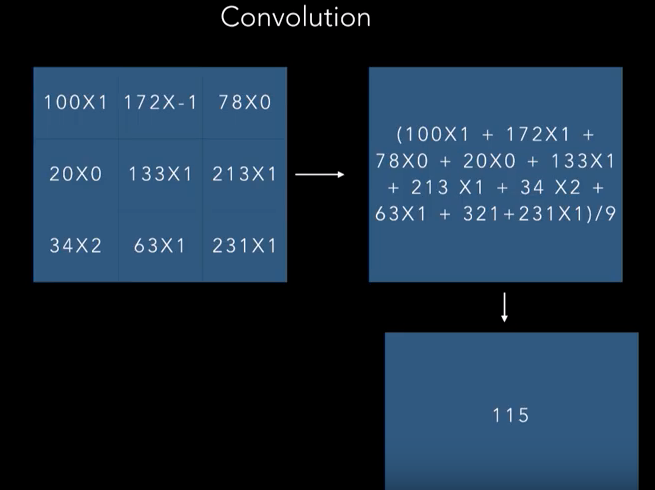Final output: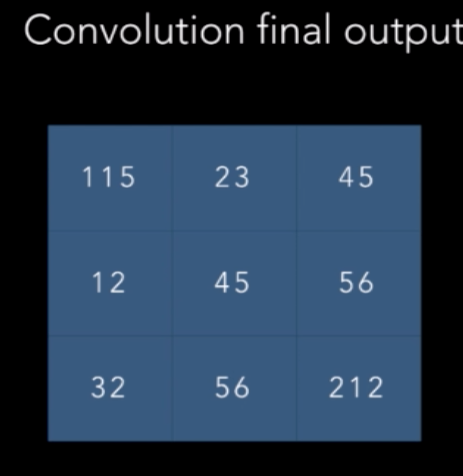As you can see a 5x5 image is reduced to 3x3 matrix after convolution. As we apply multiple convolutions in practice, it is often undesirable to have reduced matrix size. In order to avoid , we use technique called padding wherein we inflate input image size by copying borders to increae the size in such a way that post convolution image size is same as original size. For instance, if original image size is 5x5 and kernel size is 3x3, then we compute paadding as follows

padding = image size — kernel size +1 = 1

This we means we add 1 row to top and bottom and 1 column to left and right and the image size becomes 7x7. Then we apply convolution using 3x3 kenel which reduces the image size to 5x5 i.e.e same size as original.

Here is visual explanation of padding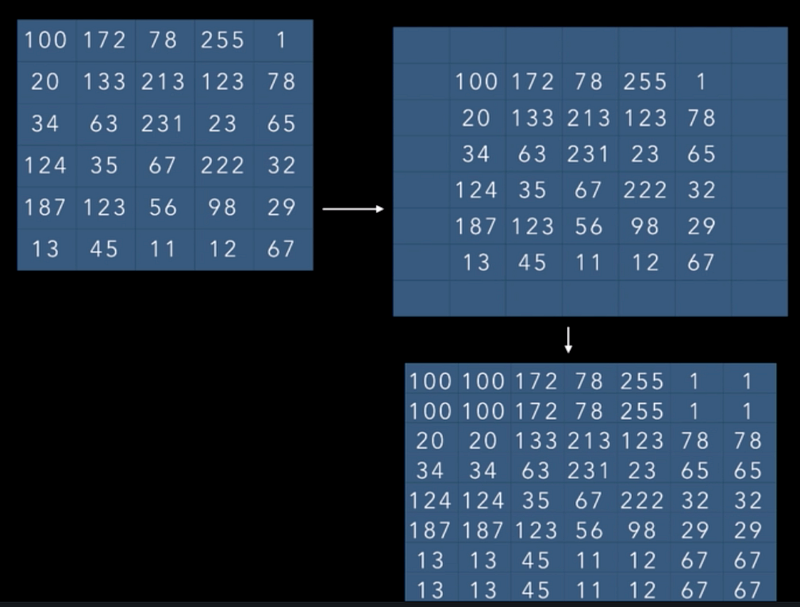Here is python code to perform convolution

```def convolve(gray, kernel):
#Get the original height and width of image
(iHeight, iWidth) = gray.shape[:2]

#Get height and width of kernel
(kHeight, kWidth) = kernel.shape[:2]

# allocate memory for the output image, taking care to
# "pad" the borders of the input image so the spatial
# size (i.e., width and height) are not reduced

pad = (kWidth - 1) // 2

#Inflate image size before applying convolution with padding so that
#image size remains same as original even after applying padding
cv2.BORDER_REPLICATE)

output = np.zeros((iHeight, iWidth), dtype="float32")

# loop over the input image, "sliding" the kernel across
# each (x, y)-coordinate from left-to-right and top to
# bottom
#slide kernel from top to bottom
#slide kernel from left to right
#get area of image which has same size of kernet
# perform element-wise multiplicate between the ROI and
# the kernel, then calculate sum
k = (roi * kernel).sum()
# store the convolved value in the output
return output```

Here are some visual effects in action using kernels. Courtesy: Setosa.ai

Here is original image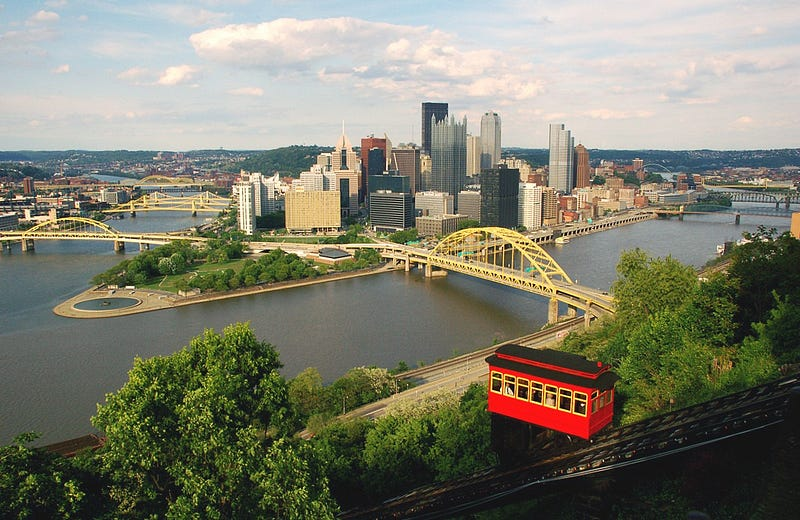We first convert it to graycsale image by applying opencv2.cvtColor function

`gray = cv2.cvtColor(image, cv2.COLOR_BGR2GRAY)`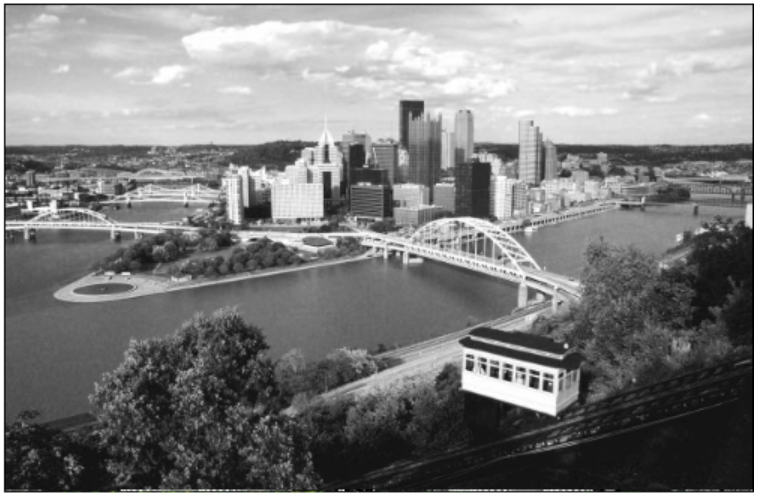Blur Kernel : Blurs the image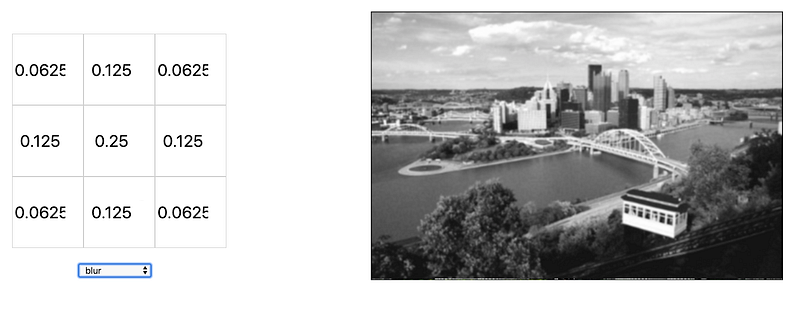Emboss Kernel : Gives perception of depth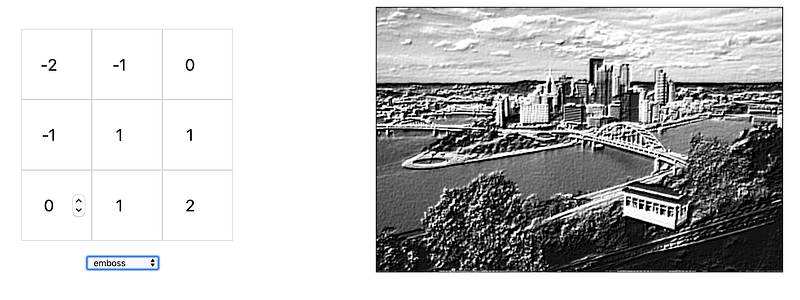Outline Kernel : Shows outlines in image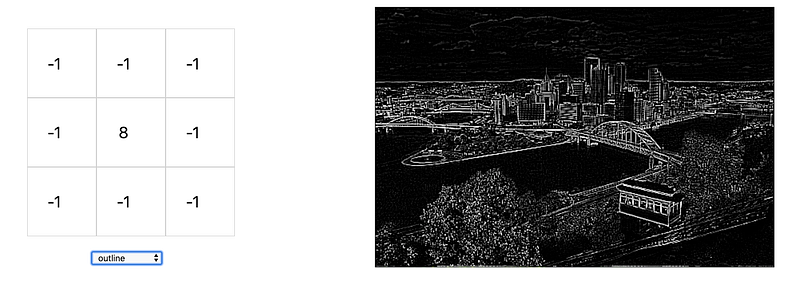Sharpen Kernel : sharpens image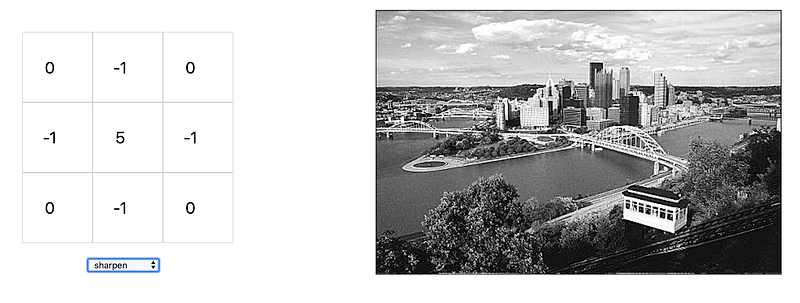Sobel Top Kernel: Highlights top part of image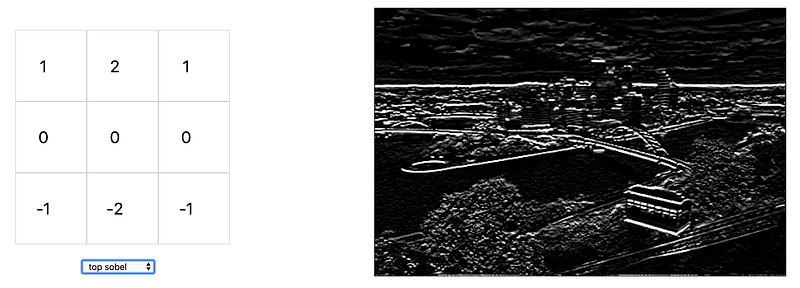So you can see and appreciate how kernels can be used effectively to manipulate image.

Some of these convolutions are effectively used for edge and outline detection in deep learning algorithms like CNN and these detections are cascaded together to form higher level interpretations like object detection.

If you would like to learn step by step on how to use image convolutions. You can sign up for full course at any of the following links

https://evergreen-school1.teachable.com/p/fundamentals-of-image-convolutions-using-opencv-an-python/?preview=logged_out

https://www.udemy.com/course/fundamentals-of-image-convolutions/?referralCode=27E0D3E7531C50F8550A

Here is introductory video

Active in teaching online courses in Computer vision , Natural Language Processing and SaaS system developmentOver 20 years of experience in fortune 500 companies

•Udemy: Evergreen Technologies

See All